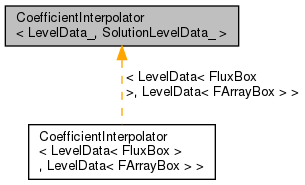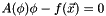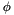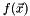Chombo + EB  3.2
CoefficientInterpolator< LevelData_, SolutionLevelData_ > Class Template Reference

#include <CoefficientInterpolator.H>

Inheritance diagram for CoefficientInterpolator< LevelData_, SolutionLevelData_ >:[legend]

## Public Types

typedef LevelData_ LevelDataType

typedef SolutionLevelData_ SolutionLevelDataType

## Public Member Functions

CoefficientInterpolator (int a_numComps)

virtual ~CoefficientInterpolator ()
Destructor. More...

int numComps () const
Returns the number of components in the interpolated coefficient. More...

virtual void interpolate (LevelDataType &a_result, Real a_time)

virtual void interpolate (LevelDataType &a_result, const SolutionLevelDataType &a_solution, Real a_time)

virtual bool dependsUponSolution () const

virtual void interpolatePrime (LevelDataType &a_prime, const SolutionLevelDataType &a_solution, Real a_time)

virtual void solve (SolutionLevelDataType &a_phi, const SolutionLevelDataType &a_f, Real a_time, const SolutionLevelDataType &a_phi0, Real a_tolerance)

void NewtonRaphson (SolutionLevelDataType &a_phi, const SolutionLevelDataType &a_f, Real a_time, const SolutionLevelDataType &a_phi0, Real a_tolerance)

## Private Member Functions

CoefficientInterpolator ()

CoefficientInterpolator (const CoefficientInterpolator &)

CoefficientInterpolatoroperator= (const CoefficientInterpolator &)

int m_numComps

bool m_inCall

## Detailed Description

### template<typename LevelData_, typename SolutionLevelData_ = LevelData_> class CoefficientInterpolator< LevelData_, SolutionLevelData_ >

This abstract base class provides an interface to time-dependent spatial coefficient data for various partial differential equations. One obtains constant access to coefficient data by specifying the desired time centering. Subclasses of this base class define how that coefficient data is computed.

Template Parameters
 LevelDataType The LevelData type used to store coefficient data.

## ◆ LevelDataType

template<typename LevelData_, typename SolutionLevelData_ = LevelData_>
 typedef LevelData_ CoefficientInterpolator< LevelData_, SolutionLevelData_ >::LevelDataType

## ◆ SolutionLevelDataType

template<typename LevelData_, typename SolutionLevelData_ = LevelData_>
 typedef SolutionLevelData_ CoefficientInterpolator< LevelData_, SolutionLevelData_ >::SolutionLevelDataType

## ◆ CoefficientInterpolator() [1/3]

template<typename LevelData_ , typename SolutionLevelData_ >
 CoefficientInterpolator< LevelData_, SolutionLevelData_ >::CoefficientInterpolator ( int a_numComps )
explicit

Base class constructor. Called by every subclass.

Parameters
 a_numComps The number of components in the interpolated coefficient.

## ◆ ~CoefficientInterpolator()

template<typename LevelData_ , typename SolutionLevelData_ >
 CoefficientInterpolator< LevelData_, SolutionLevelData_ >::~CoefficientInterpolator ( )
virtual

Destructor.

## ◆ CoefficientInterpolator() [2/3]

template<typename LevelData_, typename SolutionLevelData_ = LevelData_>
 CoefficientInterpolator< LevelData_, SolutionLevelData_ >::CoefficientInterpolator ( )
private

## ◆ CoefficientInterpolator() [3/3]

template<typename LevelData_, typename SolutionLevelData_ = LevelData_>
 CoefficientInterpolator< LevelData_, SolutionLevelData_ >::CoefficientInterpolator ( const CoefficientInterpolator< LevelData_, SolutionLevelData_ > & )
private

## ◆ numComps()

template<typename LevelData_ , typename SolutionLevelData_ >
 int CoefficientInterpolator< LevelData_, SolutionLevelData_ >::numComps ( ) const

Returns the number of components in the interpolated coefficient.

## ◆ interpolate() [1/2]

template<typename LevelData_ , typename SolutionLevelData_ >
 void CoefficientInterpolator< LevelData_, SolutionLevelData_ >::interpolate ( LevelDataType & a_result, Real a_time )
virtual

Interpolate the coefficient at the given time, placing the result in the given LevelData object. This method must be overridden by subclasses.

Parameters
 a_result The LevelData object that will store the result. a_time The time at which the coefficient is to be evaluated.

## ◆ interpolate() [2/2]

template<typename LevelData_ , typename SolutionLevelData_ >
 void CoefficientInterpolator< LevelData_, SolutionLevelData_ >::interpolate ( LevelDataType & a_result, const SolutionLevelDataType & a_solution, Real a_time )
virtual

Interpolate the coefficient at the given time, placing the result in the given LevelData object. This method assumes that the coefficient depends upon the solution to the partial differential equation in question, so the solution is passed into it as an argument.

Parameters
 a_result The LevelData object that will store the result. a_solution The solution to the partial differential equation. a_time The time at which the coefficient is to be evaluated.

## ◆ dependsUponSolution()

template<typename LevelData_ , typename SolutionLevelData_ >
 bool CoefficientInterpolator< LevelData_, SolutionLevelData_ >::dependsUponSolution ( ) const
virtual

Returns true if the coefficient depends on the solution to the partial differential equation (rendering it nonlinear), false otherwise. By default, the coefficient is assumed not to depend upon the solution.

## ◆ interpolatePrime()

template<typename LevelData_ , typename SolutionLevelData_ >
 void CoefficientInterpolator< LevelData_, SolutionLevelData_ >::interpolatePrime ( LevelDataType & a_prime, const SolutionLevelDataType & a_solution, Real a_time )
virtual

Computes the derivative of the coefficient with respect to the solution at the desired time. By default, this sets a_deriv to 0.

Parameters
 a_prime The coefficient derivative data will be stored here. a_solution The solution to the partial differential equation. a_time The time at which to compute the coefficient data.

## ◆ solve()

template<typename LevelData_ , typename SolutionLevelData_ >
 void CoefficientInterpolator< LevelData_, SolutionLevelData_ >::solve ( SolutionLevelDataType & a_phi, const SolutionLevelDataType & a_f, Real a_time, const SolutionLevelDataType & a_phi0, Real a_tolerance )
virtual

This virtual void method performs the iterative nonlinear solvefor.

Parameters
 a_phi The solution to the equation,, will be stored here. a_f The termin the equation. a_time The time at which the equation is solved. a_phi0 The initial estimate for. a_tolerance The threshold for the error in the equation that dictates when iteration should cease.

## ◆ NewtonRaphson()

template<typename LevelData_ , typename SolutionLevelData_ >
 void CoefficientInterpolator< LevelData_, SolutionLevelData_ >::NewtonRaphson ( SolutionLevelDataType & a_phi, const SolutionLevelDataType & a_f, Real a_time, const SolutionLevelDataType & a_phi0, Real a_tolerance )

This helper method solves the nonlinear equationforusing Newton-Raphson iteration. It can be used by subclasses to implement the solve() virtual method.

Parameters
 a_phi The solution to the equation,, will be stored here. a_f The termin the equation. a_time The time at which the equation is solved. a_phi0 The initial estimate for. a_tolerance The threshold for the error in the equation that dictates when iteration should cease.

## ◆ operator=()

template<typename LevelData_, typename SolutionLevelData_ = LevelData_>
 CoefficientInterpolator& CoefficientInterpolator< LevelData_, SolutionLevelData_ >::operator= ( const CoefficientInterpolator< LevelData_, SolutionLevelData_ > & )
private

## ◆ m_numComps

template<typename LevelData_, typename SolutionLevelData_ = LevelData_>
 int CoefficientInterpolator< LevelData_, SolutionLevelData_ >::m_numComps
private

## ◆ m_inCall

template<typename LevelData_, typename SolutionLevelData_ = LevelData_>
 bool CoefficientInterpolator< LevelData_, SolutionLevelData_ >::m_inCall
private

The documentation for this class was generated from the following file: